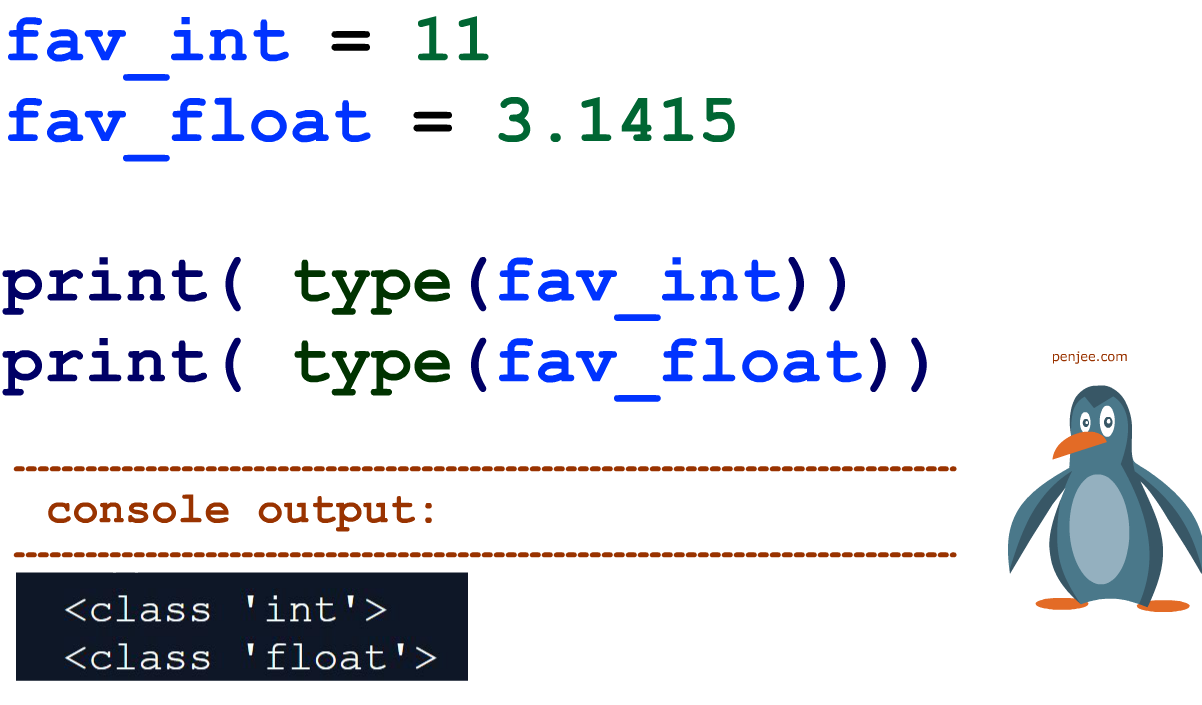## What is a Floating Point Number?

In short, a floating point number is number that represents decimal values like 3.1415

Contrast that with an integer like 11 .

# Demo Python Code

The code below declares and then prints the data type, in Python, of a floating point number and an integer.The Parts of a Floating Point Number

1. significand – the number’s actual digits
2. exponent – this exponent states where the “point” in decimal point is placed.

The idea is that the decimal point “floats” based on the value of the exponent!

The number 523.0 for example can be written in scientific notation as 523.0*10052.30*101 or 5.230*102. This example shows the “floating” decimal point which appears on different positions in the number x depending on the exponent y. Moving the decimal point one location to the right increases the exponent, moving it to the left decreases the exponent. (taken him from our article on floating point conversion)

If you’d like to mess around with representing floating point numbers, check out Float Toy.

In some programming languages, like JavaScript, all numbers are floating point numbers and there is no distinct integer datatype. However, most languages like Java or Python have 2 general classes for numeric datatypes – Integer vs Floating Point.